Home » FAQ » Why induction motors always run on lagging power factor?

# Why induction motors always run on lagging power factor?

In order to understand this mystery, I believe you will find this short recap of power factor useful. A power factor is the ratio of "real power" and the "apparent power", also listed as cosine (Theta) of the angle between real power and apparent power of power triangle, where the hypotenuse is the apparent power, the horizontal is the real power and the vertical is the reactive power. This theta angle is also the same angle between the voltage and current waveforms at any given time.

In DC system it is easy to understand that the power transfer. It is simply voltage times the current drawn by the load. However in AC systems, it is not that simple because unlike the DC system, phase angle difference between the voltage and current waveforms makes big difference in the power consumed and transferred. Let's analyze these three scenarios and compute instantaneous power by simply multiplying voltage and current waveforms.

1. Current and voltage are in sync - Instantaneous power will oscillate between max and zero, thus having a positive mean value over a given time. If a load connected to a voltage source exhibit this behavior it is said to be operating at unity power factor. Ideal resistors when connected to an AC source will have this behavior and the current flowing through resistor will produce heat (real power). In this case Real power is equal to apparent power.

2. Current lagging voltage by 90 degrees. – In this case the instantaneous power will oscillate between positive and negative max and min. However in this case the mean power over a time period will be zero. If mean power is zero, it means that no power is consumed. Ideal inductors exhibit this behavior, in other words they store the electrical energy in magnetic field without consuming it. In this case the real power is zero and the power factor is zero too.

3. Current leading voltage by 90 degrees – Similar to (2), in this case the instantaneous power will oscillate between positive and negative max and min. And also in this case the mean power consumed over a given time period will be zero. It means no real power is consumed. Ideal capacitors exhibit this behavior, in other words they store electrical energy in the form of electric fields without consuming it. In this case the real power is zero and the power factor is zero too.

Now any real component whether it is an inductor or capacitor is not ideal and has combination of resistance, inductance and capacitance. This non-linearity will create some phase difference in consumed current when connected to an AC voltage source.

Now any electric machine's stator contains windings which are basically the inductors with some inductance and resistance. So conceptually they will consume the current at a lagging power factor because the current drawn will always be lagging the voltage. Now next question one might ponder is that then how any machines say synchronous machine would be able to operate at any other power factor besides lagging? Synchronous machines unlike induction machine have coils and circuitry on the rotor which can be directly controlled to adjust the magnetic field. This control over the magnetic field makes it possible to operate the synchronous machine at any power factor. You can read more about how to do in textbooks. Induction machine has no such control and thus cannot operate at anything but lagging power factor themselves because of physics laws.

What can be done to make the induction machine run at any other power factor?

However if capacitors are added in parallel to the machine terminals, the grid do not see the same impedance it once saw when only induction machine was connected. This combined impedance of the motor and the capacitor is reflected to the grid and it changes the phase angle of the current w.r.t. to voltage when it is drawn from the utility voltage source and hence changes the net power factor measured by the utility meter. It should be noted here that the induction machine itself is still operating at the lagging power factor in this case, but the utility company or the grid won't be able to sense it.

Plz Calculate (8 - 7) =
(Your comment will show after approved.)

You may also like:

Category
Featured Articles
Non-Enclosure Variable Frequency Drive ...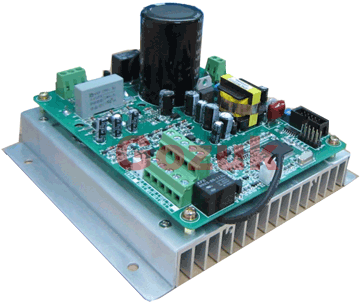No enclosure (cover), reducing installation space and cost effective. Widely used in All-In-One control cabinet. Keep the same ...
Variable frequency drive application ...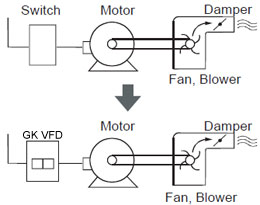Variable Frequency Drive (VFD) can be used in lots of fields. Variable frequency drives are widely used to control the speed of ...
Variable frequency drive in HVAC ...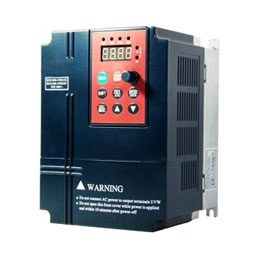Variable frequency drives (VFD) have been used for HVAC systems in buildings for more than 40 years. But only in recent years, ...
Variable Frequency Drive Harmonics and ... A discussion of the benefits of variable frequency drives often leads to a question regarding electrical harmonic distortion ...
Three phase inverters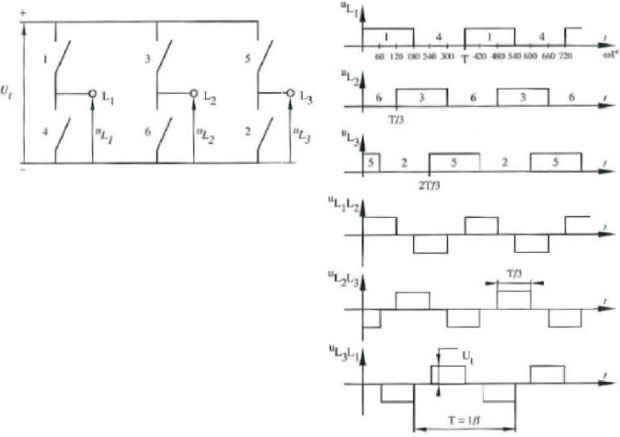In the variable frequency drive rectifier paper, it explains how to go from three phase alternating current voltage to a direct ...VFD manufacturers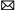buy@vfds.orgtech@vfds.org